# The Programmatic Side of Mathematica V: Segue into Primes

You might recall in the last post the expansion of a general binomial$(a + b)^z$:

binexpand[z_] := Expand[(a + b)^z]
TableForm[Array[binexpand,6]]

You can also declare a loop in the way Mathematica does so that it gives a series of binomial expansions. Below is the output of the second statement from above, the first 6 expansions of a general binomial:

And if you want to mess around with the Map command, you can put square roots on everything:

You don’t always need to use Array[] to generate a list. You can also use Range[hi] which generates a list from 1 to “hi”:

Prime[Range]

Generates the first 100 primes:

{2, 3, 5, 7, 11, 13, 17, 19, 23, 29, 31, 37, 41, 43, 47, 53, 59, 61, 67, 71, \
73, 79, 83, 89, 97, 101, 103, 107, 109, 113, 127, 131, 137, 139, 149, 151, \
157, 163, 167, 173, 179, 181, 191, 193, 197, 199, 211, 223, 227, 229, 233, \
239, 241, 251, 257, 263, 269, 271, 277, 281, 283, 293, 307, 311, 313, 317, \
331, 337, 347, 349, 353, 359, 367, 373, 379, 383, 389, 397, 401, 409, 419, \
421, 431, 433, 439, 443, 449, 457, 461, 463, 467, 479, 487, 491, 499, 503, \
509, 521, 523, 541}

In earlier posts I had been curious about the distribution of prime numbers along the number line, and took my research to very large numbers, but I fear not large enough since I don’t have a supercomputer handy. My considered opinon first-hand, is that the primes are initially densely distributed, then gets gradually sparser in a way that is hard to predict. Mathematica claims it can easily access primes as high as on the order of$10^8$ in magnitude, and likely does the higher primes more slowly due to the fact that it uses primes up to that magnitude to search for still higher primes. If a natural number n has no prime numbers as factors, then n itself is prime.

The Mersenne primes, those Mersenne primes which are still being sought after, numbers large enough to fill every page in a 200-page novel, are notoriously hard to prove indivisible, since I would fear that the primes up to$10^8$ are not good enough. You need higher primes still, to many more orders of magnitude. Knowing the previous Mersenne prime is unreliable, since who knows how many primes lay between the current prime being checked and the previous one? There are likely to be hundreds, or even thousands of undiscovered primes which lay between$2^{p_{n-2}} - 1$ and$2^{p_{n-1}} - 1$, assuming$2^{p_{n-2}}$ and$2^{p_{n-1}}$ are prime (p, the exponent, is prime as well). The method of last resort, as I understand it, is to try every number up to$\lfloor \sqrt{2^{p}} \rfloor$ past$10^8$. While trying primes below$10^8$ weeds out several numbers, I think would still take a very long time for certain numbers that remain unfactored past that, possibly days to months even on a fast computer processor — to weed out one number or to successfully prove it as prime.

All other prime numbers which lay between two consecutive Mersenne primes (especially the big numbers) are nearly impossible to find, and I am not aware of anyone who bothers trying to find them (that doesn’t mean no one is trying, though). To find the next prime up from a Mersenne prime means looking to the next consecutive odd number, and the next, and the next, until you find one. A lot of time — months of time — is possibly wasted on a lot of composite numbers. Mersenne numbers narrow the search down, but only to a certain kind of prime number.

Mathematica has, for what it’s worth, a function called Prime[n], which returns the nth prime. Prime returns 2; Prime returns 3, and so on. The 100th prime is 541:

Prime
541

And if we subtracted

Prime - Prime
539

we can say that 539 integers lay between the first and the 100th prime. We can now do this:

Prime - Prime
1606

which tells us there is a difference of 1606 between the millionth prime and the 1,000,100th prime.

Prime - Prime
1974

The air gets thinner, but only slightly, when counting between the billionth prime and the 1,000,000,100th prime. By the trillionth prime, we get a difference of 3546 by the 1,000,000,000,100th prime. Or you can say 100 primes per 3546 consecutive integers. The trillionth prime is 29,996,224,275,833, or just under 30 trillion. Mathematica’s Prime[] function chokes when getting to numbers on the order of a quadrillion, even though the documentation claims arguments to Prime[] are allowed to go into the quintillions: 1,152,921,504,606,846,976 or 260.

I could say that this still provides an incredible list of primes for the possible writing of a prime factorization function, however, Mathematica has beat me to it, wtih the function FactorInteger[]. Even the Mathematica website at Wolfram Math World has defined a function that modifies the output of this function further:

FactorForm[n_?NumberQ,fac_:Automatic]:=
Times@@(HoldForm[Power[##]]&@@@FactorInteger[n,fac])

So, a call to FactorForm[] can be:

I don’t know what “Times@@” does, but I don’t seem to get anything other than the default terminal font. At any rate, the ordered pairs returned by FactorInteger[] are transformed into base integers and exponents.

# The Mersenne Prime Search

I have recently joined GIMPS, and have installed their prime search software on two of my computers.

Their website is at: mersenne.org.

# The Programmatic Side of Mathematica II

Since the last time I posted, I found a better way to do 100 dice rolls: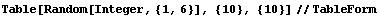This gives 100 dice rolls in a tabulated format in a much simpler syntax. You can also make a “die” out of complex numbers, but it is a little more of an issue to truncate: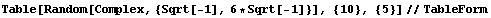… which gives output like: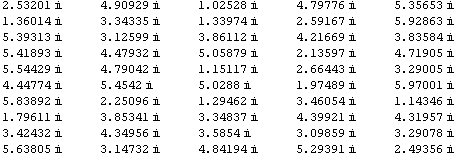Strictly speaking, these are all a kind of complex number called a pure imaginary number, but I guess I can’t split hairs.

You can create a list of divisors of integers like 28, like so:

Divisors
{1, 2, 4, 7, 14, 28}

28 is a called a perfect number, because like 6, the number can be created by the sum of its proper factors. A “proper factor” of a number k does not include k itself, so if you add the numbers generated in the above list, you will obtain 2k, or 56.

So to get the correct result, you can make your own function that can re-generate the list with the last element truncated. The Drop[] function can do this: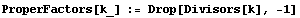I have declared a small function called ProperFactors, and the underscore after the k indicates that it is a placeholder for a parameter. When you run this, it returns nothing, but of course all you really did was declare a function. The Drop[] function drops elements from the list generated by the Divisors[] function, beginning at the last element (the reason there is a “-1” passed to Drop[]. In the list generated before, the last element was the highest factor of 28, which was 28 itself. The remaining elements in the list are the proper factors.

So, the call:

ProperFactors

generates the list:

{1, 2, 4, 7, 14}

which are the proper factors of 28. Let’s programmatically check to see if the sum of the proper factors add to 28. Of course, checking by adding the numbers up yourself will tell you this: 1 + 2 + 4 + 7 + 14 = 28. But making a function do the work will be beneficial if the factors become very large. So, we can make use of the Apply[] function:

Apply[Plus, {1,2,3,4,5}]

This returns:

15

or the sum of 1 + 2 + 3 + 4  + 5. It takes a list of numbers (an actual array), and treats them as though each element were parameters passed into the Plus[] function. I could get the same thing with:

Plus[1, 2, 3, 4, 5]
15

The curly braces aren’t needed, since Plus[] expects a list of arbitrary length, but not an array. Since ProperFactors[] generates an array, I need to convert the array into a list to be operated on by Plus[]. This is the reason for the Apply[] function.

List[] is a function which generates an array:

List[1,2,3,4,5]
{1, 2, 3, 4, 5}

And simply invoking the literal array {1, 2, 3, 4, 5} does the same thing as List[]. And you can assign the array to a variable.

s := List[2, 3, 4, 5, 6]

does the same thing as

s:= {2, 3, 4, 5, 6}

so that

Apply[Plus, s]

converts the array s to a list of parameters passed to Plus. The result is:

20

Now, let’s go back to our perfect number checking function. Let’s call it PerfectCheck[]:

PerfectCheck[k_] := Apply[Plus, ProperFactors[k]] == k

What does “Apply[Plus, ProperFactors[k]]” do? Let’s invoke that by itself in Mathematica to see, using k = 28:

Apply[Plus, ProperFactors]
28

so, it adds up the proper factors of 28. To make sure, let’s use a non-perfect composite number:

Apply[Plus, ProperFactors]
117

To check this, go further back and list the proper factors of 100 themselves: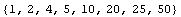You will see when adding by hand that these add to 117.

Thus, a PerfectCheck would be false since 117 <> 100.

PerfectCheck
false
PerfectCheck
true
PerfectCheck
true
PerfectCheck
false

Checking numbers in the range 1 to n can be done using Select[]:

perfect[n_] := Select[Range[n], PerfectCheck]

So that:

perfect

yields:

{6, 28, 496, 8128}

I was trying to find a fifth perfect number, but it’s difficult. I even cranked up the call to perfect but no luck, with Mathematica chugging away for about 10 minutes. But I was able to find information through Google more quickly. According to The Math Forum, they say that the fifth one was still some ways off: 33,550,336.

A sixth number may exist, but as you can see the trend seems to indicate that they are pretty sparse on the number line. The Math forum hinted that these perfect numbers obey a number pattern according to the formula: (2n)(2n+1 – 1). This isn’t really reliable; that is to say that the value of integer used for n will not generate the nth perfect number necessarily. This seems to generate a perfect number so long as 2n+1 – 1 equals the value of a Mersenne Prime. A Mersenne Prime is a prime number that is one less than some power of 2, such as the number 7, which is 23 – 1. When finding the “highest known prime number” these days, mathematicians look for Mersenne Primes.

Using a command like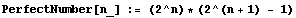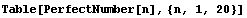can fool you into thinking you have just printed out the first 20 perfect numbers, but you would be wrong. The list it gives out: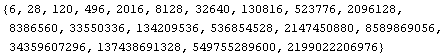has numbers, such as 120, 496, and 2016, that are not perfect. You still need to check each one with our PerfectCheck[] function (either that, or fish out the numbers with Mersenne prime factors among the lot).

You would have to keep hunting until you find 8,589,869,056 as being the sixth perfect number, closer to the end of the list. Then the seventh one is two more over: 137,438,691,328.

But, of course we have a computer, so life gets happy once again for the slack among us. We can mash up the Table[] call above, and Select[] numbers that are perfect with PerfectCheck: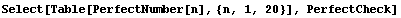which generates the array: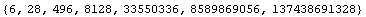and all of these are perfect numbers. I can go even more nutzo and increase the upper limit to 100: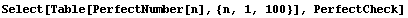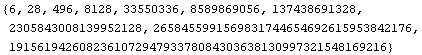… thereby generating the first 10 perfect numbers.

There are to date only 47 known Mersenne primes according to Wikipedia. Therefore, there are only 47  known perfect numbers, assuming all perfect numbers are even.